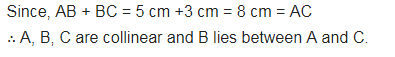# If A, B, C are three points on a line such that AB = 5 cm, BC = 3 cm and AC = 8 cm,

If A, B, C are three points on a line such that AB = 5 cm, BC = 3 cm and AC = 8 cm, which one of them lies between the other two?